### Testing Lithium Ion Batteries

Purpose of This Note

This application note discusses electrochemical measurements on lithium ion batteries. Theory and general setup of lithium ion batteries are explained. Important parameters for characterizing batteries are described.

In addition, various experiments on coin cells are performed. They show how to gain information about a battery’s performance, e.g. capacity and voltage limits as well as long time behavior.

Introduction

Batteries are indispensable energy storage systems for mobile and stationary applications. They are mostly used for portable devices or when electrical lines are impractical or impossible.

Their application fields reach from smaller devices such as MP3 players or smartphones up to high power systems for the automotive market or energy storage systems for power plants, e.g. wind power plants.

Setup

The typical setup of batteries consists of two oppositely charged electrodes which are separated by an electrolyte. They can be classified as primary or secondary cells, depending on their chemical system.

Primary cells

Primary batteries can not be recharged. They are already fully charged and can be used immediately. They offer high specific energies and long storage times.
However, primary cells cover currently only a niche market. They are in general used when rechargeable batteries are impractical or charging is not possible, e.g. watches, toys, or pacemaker. Other applications fields can be found in the military, e.g. missiles.

Typical primary cells are alkaline manganese, zinc-carbon or lithium batteries.

Secondary cells

In contrast to primary cells, secondary batteries can be recharged hundreds of times. Their market share increases steadily.

The oldest rechargeable battery is the lead acid battery which is still used as starter battery in vehicles or for back up systems. Other examples are nickel cadmium (NiCd), nickel metal hydride (NiMH), or lithium ion batteries. Latter one is currently in the focus of research as it is the battery of choice for the automotive market.

Figure 1 shows a typical setup of a lithium ion battery and outlines the electrochemical process during discharging.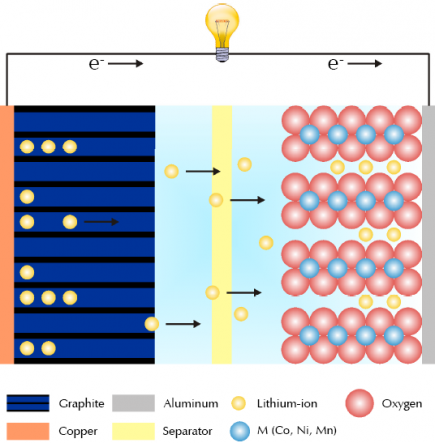Figure 1 – Simplified diagram of a lithium ion battery during discharging.
For details, see text.

In order to reach high power and energy densities, highly porous materials are used as electrode materials. For the anode, graphite is attached on copper foil which serves as current collector. Cathodes use mostly lithium transition metal oxides which are attached on aluminum foil.

The electrolyte carries out the charge transport between both electrodes. It can be liquid, solid, or polymeric. A separator – an ion permeable membrane – is placed between both electrodes to avoid electrical shorts.

During the charging process, lithium ions migrate from the lithium rich cathode to the anode and intercalate into its multi layered structure. This electrochemical process is reversed during discharging. Following chemical equations summarize both processes whereby the forward reactions illustrate the charging step.

Anode: xLi+ + xe- + C6 ⇆ LixC6
Cathode: Lix+yMO2 ⇆ xLi+ + xe- + LiyMO2

Performance and lifetime of lithium ion batteries depend strongly on several parameters. Extreme temperatures can lead to material deterioration. Exceeding the rated specifications of batteries, e.g. potential or charge and discharge current, can lead to irreversible reactions and overheating. The battery’s overall performance can be drastically reduced.

Hence voltage and current have to be monitored and controlled when charging and discharging single batteries and battery stacks. The following section discusses by means of experiments the electrochemical behavior of lithium ion batteries. The effect of different measurement parameters is shown.

Experimental

Measurements for this application note were performed on rechargeable coin cells from Great Power Battery (model LIR2032). The batteries were placed into Gamry’s battery holder for CR2032 coin cells (see Figure 2). The holders allow precise measurements due to direct contact Kelvin sensing.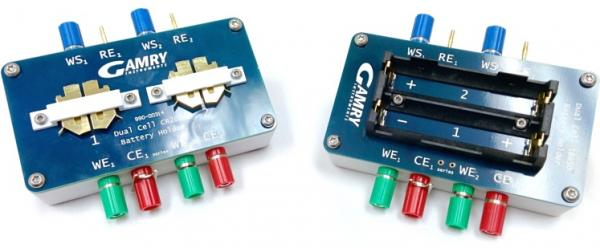Figure 2 – dual cell CR2032 (left) and 18650 battery holder (right).

All measurements were performed with an Interface 1000 potentiostat.

Charge and discharge curve

Figure 3 shows a typical charge (green) and discharge curve (blue) of a coin cell. Voltage (darker colored) and current (lighter colored) are plotted versus time. The cell was charged and discharged with a current of ±40 mA between 2.75 V and 4.2 V.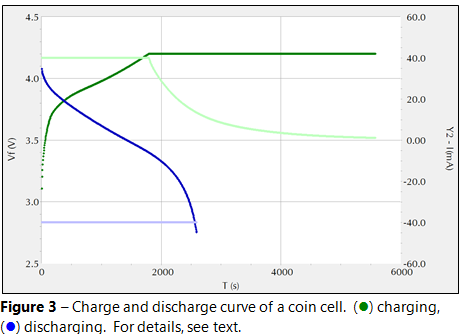Voltage increases steadily while charging the battery. During this step, lithium ions are extracted from the cathode and intercalate into the anode’s graphite layers.

The cell is potentiostatically held at 4.2 V after reaching the upper voltage limit. This step lasts until the current reaches 0.4 mA which corresponds to a C rate of 0.01. This ensures that the battery is fully charged. The battery’s state of charge (SOC) is 100 %.

The voltage initially drops at the beginning of the discharge step. According to Ohm’s law, this voltage drop ∆U (also called “IR drop”) is directly proportional to the Equivalent Series Resistance (ESR), see equation 1.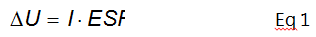I is the applied current. The ESR sums up resistances from electrodes, electrolyte, and electrical contacts. The lower the voltage drop U the higher is the maximum output energy E that can be drawn from a battery, see equation 2.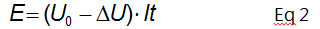U0 is the actual voltage of the battery and t the charge or discharge time respectively.
The limit of a battery’s usable capacity is reached when the voltage declines sharply. The discharge step is stopped at 2.75 V. At this potential, the SOC is defined to be 0 %. The depth of discharge (DOD) is 100 %.

Potentials that exceed the specifications of a battery should be avoided. Electrolyte decomposition or degradation of electrode materials can lead to reduced battery performance and lifetime.

C rate

The term C rate describes how fast a battery is charged or discharged. The batteries used for this application note have a rated capacity Q of 40 mAh for a 0.2 C rate. This means that ideally 8 mA can be drawn for five hours according to the following equation.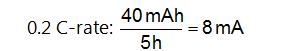Batteries can be charged faster when using higher C rates. Vice versa, energy can be drawn in a shorter period of time. However, higher C rates can drastically affect the performance and lifetime of a battery.

Figure 4 shows five discharge curves with increasing C rates (from dark to light green). The battery’s potential is plotted versus capacity. It is automatically calculated by Gamry’s Echem Analyst.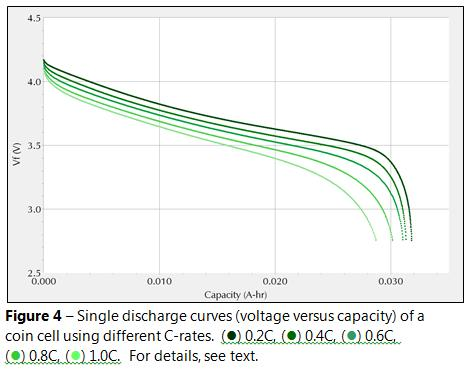The coin cell was first charged to 4.2 V and held at this potential for a longer period to fully charge the battery. Afterwards, the battery was discharged to 2.75 V. The C rate was varied between 0.2 C (8 mA) and 1.0 C (40 mA).

Table 1 summarizes several parameters which were obtained from this experiment.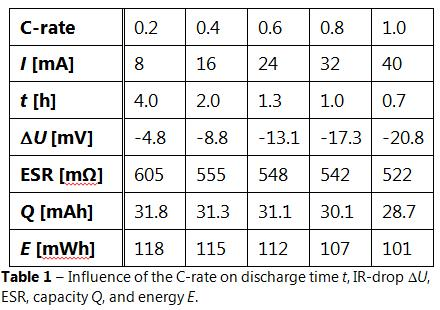As mentioned before, discharge time t decreases with increasing C rate. Note that t is shorter than the theoretical discharge time. These variations are mainly influenced by age and amount usage of a battery as well as temperature.

Increasing C rates increase also the IR drop. This affects negatively capacity and energy. Capacity is decreasing by about 10 % when increasing the C rate from 0.2 C to 1.0 C.

Note also that the ESR is decreasing with higher C rates. This can be explained by increasing temperatures within the battery. However, drawbacks like lower capacity and energy outweigh this advantage. In addition, higher temperatures can also lead to material deterioration.

Battery cycling

A typical experiment for testing a battery’s long term stability is cycling. For this, batteries are charged and discharged several hundreds of times and the capacity is measured.

Figure 5 shows a standard cycling charge discharge (CCD) experiment for batteries. The coin cell was first charged to 4.2 V with a 1.0 C rate (40 mA). This potential was then potentiostatically held for at least 72 hours or if the current reached 1 mA. The battery was then discharged with a 1.0 C rate to 2.7 V. This sequence was repeated for 100 cycles.

The darker curves show the capacity. The lighter curves show the percentage amount of capacity in relation to the beginning.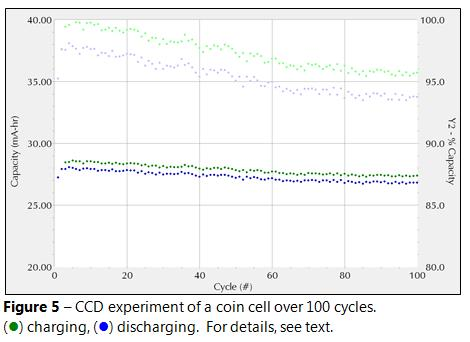Electrolyte impurities or electrode imperfections cause always loss of capacity.  The tested battery in this example shows a good cycle behavior.  The maximum capacity of the coin cell is about 28.7 mAh.  Capacity decreases only slightly after 100 cycles.  The total capacity loss sums up to about 4.5 %.

Extreme temperatures, over‑charging or over‑discharging can accelerate capacity loss. Generally, batteries should be replaced when the capacity loss is more than 20 %.

In addition, the Echem Analyst enables calculation of the Coulombic efficiency hC.  It describes the charge ratio during discharging and charging (see also equation 3).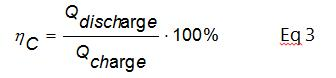The coin cell in this experiment exhibits a Coulombic efficiency of about 98 %.

Leakage current and self discharge

Ideally, a battery’s potential is constant when no external current flows. However, in reality the potential decreases with time even if a battery is not connected to an external load.

This effect is called self discharge. All energy storage devices are more or less affected by self discharge (SD).

Figure 6 shows a diagram of a self discharge experiment with a new coin cell. The battery was first charged to 4.2 V and then potentiostatically held at this potential for three days. The open circuit potential was then measured for nine days.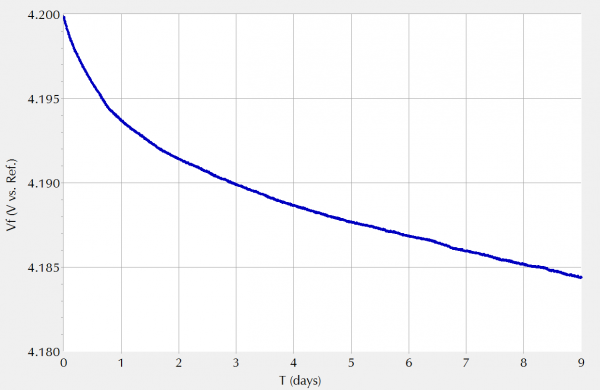Figure 6 – Self discharge experiment of a coin cell. For details, see text.

The battery shows a very good self discharge behavior. Initially, the potential decreases by more than 6 mV. Afterwards, the rate slows down to less than 1 mV per day. After nine days, the potential decreased by 15.6 mV in total. This corresponds to a potential drop only 0.37 % in relation to the initial value. Table 2 summarizes the results of the self discharge experiment.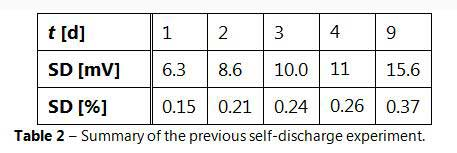Self discharge is caused by internal current flow which is called leakage current (/leakage). The rate of self discharge is mainly influenced by age and usage of a battery, its initial potential as well as temperature effects.

Figure 7 shows leakage current measurements on two coin cells. One battery was new and the other one was heated up to 100°C for a short time. Both batteries were initially charged to 4.2 V. The potential was then held constant and the current was measured.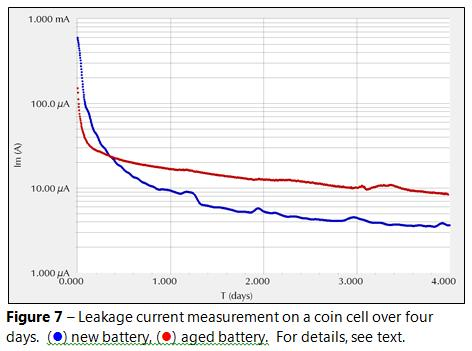The measurement was done with a special script called PWR Leakage Current.exp. It avoids I/E converter range changes by using a user entered ESR value. It is recommended not using a potentiostatic test for measuring leakage currents.

The measured current is continuously decreasing. Note that it is still not constant even after four days. However, many manufacturers specify /leakage as the value that is measured after 72 hours. In this case, leakage current is about 4.7 µA for the new battery. The aged coin cell shows with 10 µA a value which is twice as big.

In general, batteries that are not used for a longer period should be periodically checked and charged. Self discharge should not exceed 40 % as battery performance and lifetime are extremely affected. Batteries with high self discharge rates should not be used anymore.

EIS measurements

Figure 8 shows four different Nyquist diagrams at various potentials. A coin cell was first charged to 3.9 V, 4.1 V, 4.3 V, and 4.5 V respectively. The potential was then potentiostatically held until the current dropped below 1 mA. This step ensures that the potential is constant during the EIS experiment.

Galvanostatic EIS experiments were performed between 100 kHz and 10 mHz. The DC current is zero and the AC current was set to 10 mArms.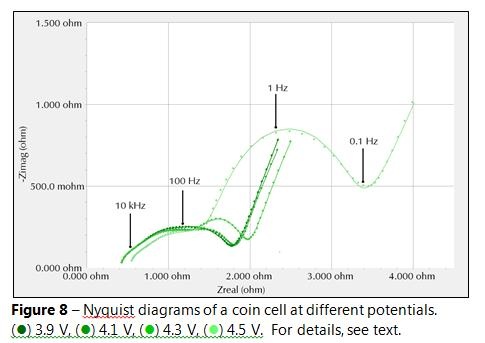The shape of the Nyquist plot depends strongly on the potential of the battery. At lower potentials, i.e. 3.9 V and 4.1 V, both curves nearly overlap.

The battery’s impedance is increasing at higher potentials. The Nyquist curves at 4.3 V and 4.5 V respectively are shifted to the right and the semi circles are bigger.

For a better understanding, EIS circuit models can be used. Figure 9 shows a typical model for lithium ion batteries.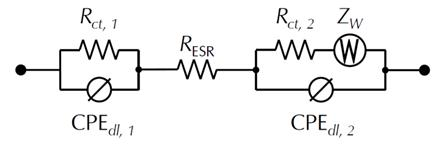Figure 9 – Simple EIS model representing a lithium ion battery. For details, see text.

RESR represents the ESR of the battery. It is the limiting impedance at high frequencies. It can be easily estimated as intersection between Nyquist curve and x axis (Zreal).

Further, it is assumed that each electrode/electrolyte interface has a double layer capacitance and a charge transfer resistance Rct. Each parallel circuit of these elements represents a semi circle in the Nyquist diagram.

In order to address porosity and inhomogeneities of both electrodes, the double layer capacitance is replaced by a constant phase element (CPE). It sums up polarization effects of the double layer at non ideal electrode/electrolyte interfaces. Ideally, CPEs can be treated as capacitors.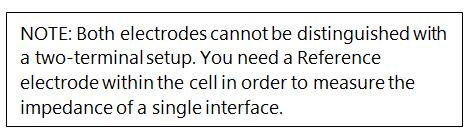All Nyquist curves show a diagonal line at an angle of 45° at low frequencies. This region can be modelled by a Warburg impedance ZW. It describes linear diffusion for inifite thickness of the diffusion layer. To simplify matters, diffusion is taken into consideration only on one electrode.

Table 3 summarizes all fit parameters that were gained from the previous EIS experiment shown in Figure 8.

Note that the parameters Y and its dimensionless exponent a define the constant phase element. Y has the unit S•sa (Siemens times second to the power of a).

For a = 1, Y has the unit Farad (F) and represents an ideal capacitor. In contrast, if a = 0, Y is the reciprocal of a resistor with the unit S = Ω-1.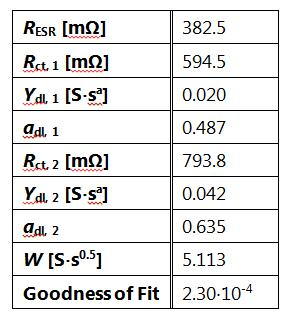Table 3 – Fit parameters of an EIS experiment performed on a coin cell charged to 3.9 V. The circuit model is shown in Figure 9.

In addition, the “Goodness of Fit” is shown which gives a good estimation if the used fit is appropriate for the current system. A value of 1.10-4 or lower indicates a very good fit. The error between measured and calculated values is only about 1 %. If the value is higher than 0.01, a different fit model should be taken into consideration.

Battery stacks

For high power applications, single cells are assembled in serial and parallel setups. Serial setups are used in applications where higher voltages are required. The total voltage U is the sum of the voltage Ui of each single battery.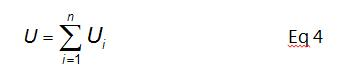In contrast, parallel setups are used when higher currents are needed. Further, batteries with lower ampere hour ratings can be used. The total current I is the sum of each battery’s single current Ii. The total voltage of the stack stays the same.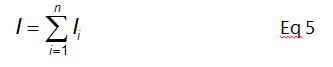Both configurations can be also combined allowing higher flexibility with standard cells. However, for battery stacks it is all the more important to avoid cell failures. Single cell failures can decrease the performance of a whole battery stack.

In general, a stack and its single cells have to be balanced. Each single cell should exhibit similar parameters, e.g. voltage window or impedance.
In unbalanced stacks, single batteries can overheat due to over charging or over discharging. Hence advanced software has to control each single battery and the whole stack.

Gamry enables monitoring cell stacks by using either multichannel potentiostat systems or the Reference 3000 with optional Auxiliary Electrometer. Both systems enable all previously discussed experiments with battery stacks. As a result, information about a cell stack as well as each single battery can be gained.

Conclusion

This application note addresses measurements with lithium ion batteries. Setup and important parameters of lithium ion batteries are explained for single batteries as well as battery stacks.

Different experiments are described by means of measurements on single coin cells. Cyclic charge discharge, leakage current, and self discharge tests are performed. Data evaluation of impedance measurements is shown by using a simple EIS model.